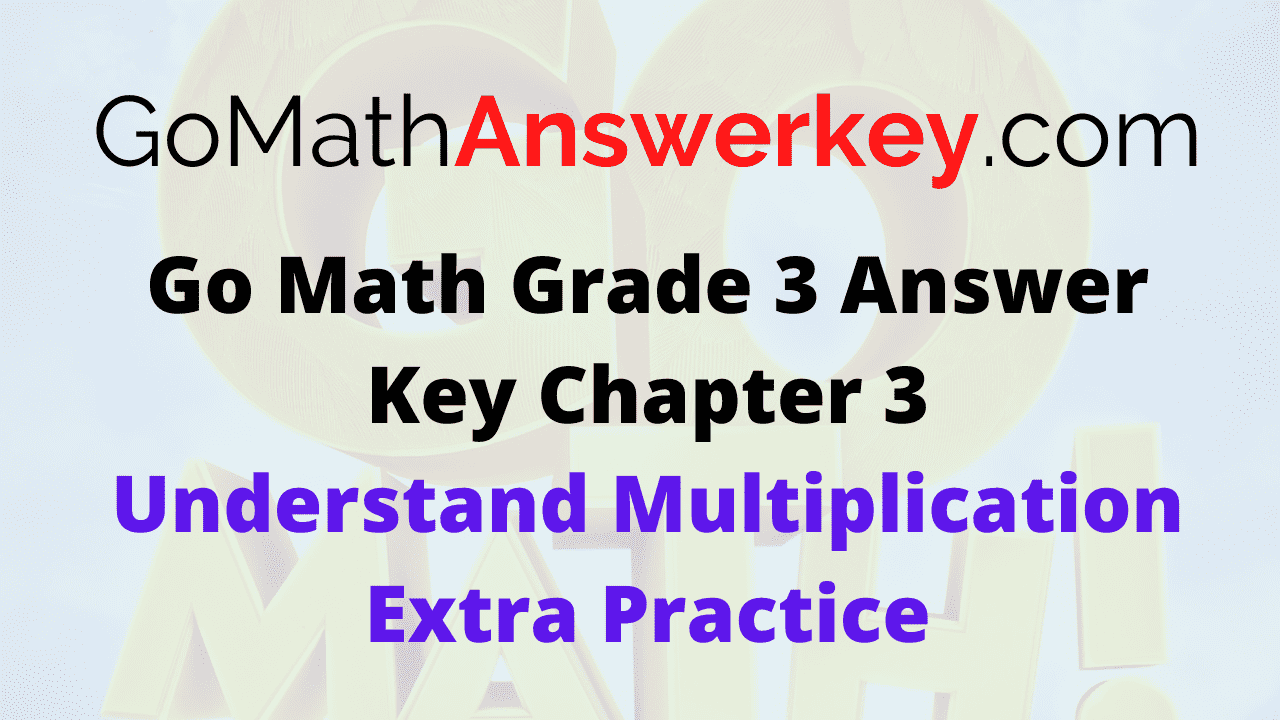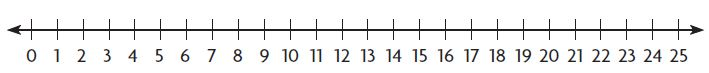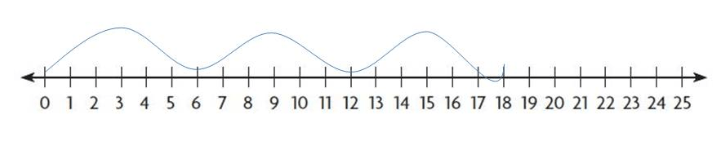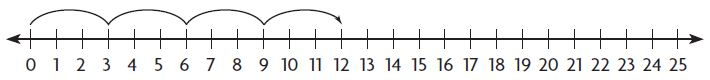# Go Math Grade 3 Answer Key Chapter 3 Understand Multiplication Extra Practice

Get the source of Go Math Grade 3 Answer Key Chapter 3 Understand Multiplication Extra Practice. All the problems are solved and included with answers and explanations. Refer Go Math Grade 3 Chapter 3 Understand Multiplication Extra Practice Answer Key for an easy process of learning. Students can easily familiar with the types of questions and solving processes with extra practice. Score high marks by solving HMH Go Math Grade 3 practice questions. 100% free resources with an excellent explanation is available on Go Math Grade 3 Extra Practice Answer Key.

## Go Math Grade 3 Chapter 3 Understand Multiplication Extra Practice Answer Key

The series of math problems and solutions will real solve practice to gain good grades. Every problem is explained with real term examples. Refer our Go Math Grade 3 Answer Key Chapter 3 Understand Multiplication Extra Practice and be on top to get a good score.

### Common Core – Page No. 63000

Lesson 3.1

Draw equal groups. Skip count to find how many.

Question 1.
2 groups of 4 ______

8

Explanation:
Draw 4 counters in each group.
There are 2 equal groups.
skip count by 4 until you say 2 numbers.
There are 2 equal groups with 4 counters in each group.
So, there are 8 counters in all.

Question 2.
4 groups of 3 ______

12

Explanation:
Draw 3 counters in each group.
There are 4 equal groups.
skip count by 3 until you say 4 numbers.
There are 4 equal groups with 3 counters in each group.
So, there are 12 counters in all.

Lesson 3.2

Draw a quick picture to show the equal groups. Then write related addition and multiplication sentences.

Question 3.
2 groups of 5
______ + ______ = ______
______ × ______ = ______

5 + 5 = 10
2 x 5 = 10

Explanation:
Draw 5 counters in each group.
There are a total of 2 groups.
Now, the addition sentence is 5 + 5 = 10.

Multiplication sentence
Draw 5 counters in each circle or group.
Since there is the same number of counters in each group, multiply counters and groups to find how many there are altogether.
2 x 5 = 10.
factor x factor = product

Question 4.
3 groups of 2
______ + ______ + ______ = ______
______ × ______ = ______

2 + 2 + 2 = 6
3 x 2 = 6

Explanation:
Draw 2 counters in each group.
There are a total of 3 groups.
Now, the addition sentence is 2 + 2 + 2 = 6

Multiplication sentence
Draw 2 counters in each circle or group.
Since there is the same number of counters in each group, multiply groups and counters to find how many there are altogether.
3 x 2 = 6.
factor x factor = product

Lesson 3.3

1. Draw jumps on the number line to show 3 groups of 6.
Find the product.

Question 5.3 × 6 = ______Explanation:
3 x 6 = 18

Write the multiplication sentence the number line shows.

Question 6.______ × ______ = ______

4 x 3 = 12

Explanation:
1 jump on the number is considered as 1 group.
There are 3 jumps on a number line. So, there are 3 groups.
The length of each jump is 4.
Begin at 0. Skip count by 3’s.
Multiply 4 x 3 = 12.

### Common Core – Page No. 64000

Lesson 3.4

Question 1.
Destiny placed her hair ribbons in 3 groups of 5 on her dresser. How many hair ribbons in all does Destiny have? Draw a diagram to solve.
______ hair ribbons

15 hair ribbons

Explanation:
3 groups of 5
Draw 3 counters (hair ribbons) in each group.
There are 5 equal groups (dresser).
We skip count by 3’s until you say 5 numbers (3,6,9,12,15)
There are 15 hair ribbons in all.

Lesson 3.5

Draw an array to find the product.

Question 2.
2 × 7 = ______

2 x 7 = 14

Explanation:2 x 7 = 14

Question 3.
2 × 6 = ______

2 x 6 = 12

Explanation:2 x 6 = 12

Lesson 3.6

Write a multiplication sentence for the model. Then use the Commutative Property of Multiplication to write a related multiplication sentence.

Question 4.
□□□□□
□□□□□
□□□□□
□□□□□
______ × ______ = ______
______ × ______ = ______

4 x 5 = 20
5 x 4 = 20

Explanation:
The given image has 4 rows and 5 columns of square boxes. So, multiplication = 4 x 5. Using Commutative Property of Multiplication 4 x 5 = 5 x 4.

Question 5.
______ × ______ = ______
______ × ______ = ______

Explanation:

Lesson 3.7

Find the product.

Question 6.
6 × 0 = ______

0

Explanation:
The Zero Property of Multiplication states that the product of zero and any number is zero. So, 6 x 0 = 0.

Question 7.
5 × 1 = ______

5

Explanation:
Use Commutative Property of Multiplication: 5 x 1 = 1 x 5.
The Identity Property of Multiplication states that the product of any number and 1 is that number. 5 x 1 = 5.

Question 8.
0 × 9 = ______

0

Explanation:
The Zero Property of Multiplication states that the product of zero and any number is zero. So, 0 x 9 = 0.

Question 9.
1 × 8 = ______

8

Explanation:
The Identity Property of Multiplication states that the product of any number and 1 is that number. 1 x 8 = 8.

Question 10.
1 × 4 = ______

4

Explanation:
The Identity Property of Multiplication states that the product of any number and 1 is that number. 1 x 4 = 4.

Question 11.
9 × 1 = ______

9

Explanation:
Use Commutative Property of Multiplication: 9 x 1 = 1 x 9.
The Identity Property of Multiplication states that the product of any number and 1 is that number. 9 x 1 = 9.

Question 12.
1 × 0 = ______

0

Explanation:
The Zero Property of Multiplication states that the product of zero and any number is zero.
So, 1 x 0 = 0.

Question 13.
7 × 0 = ______